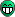# Use cellphone number saved on the SD card to send SMS

Hello, how are you?

One of the parts of my application comes down to open an existing .txt file on the SD card, read your content, which refers to a cell number, and use that number read from the card file for a message to be sent to it .
With the example exist in the library can read smoothly the number on the SD card, and the library Linkit_AT you provided have also successfully send SMS and make calls, my problem is when I will write the value read from the SD card file, int celSD myFile.read = (); , In the phone number of the variable for messaging, LSMS.beginSMS (numCel); I made up all kinds of conversion using tools such as sprintf () and itoa () without success, I’m making a mistake in converting the variables and do not know what, I understand I read an int value and must convert to a char [] however, when I do this is shown only the last number of the cell and not the whole number, as if I was just reading the last byte of the array, can you help me?Thank you!

This is the part of my code:

myFile = LSD.open(“celular.txt”);
if (myFile) {
Serial.println(“celular.txt aberto”);
myFile.seek(0);
// read from the file until there’s nothing else in it:
while (myFile.available()) {
// numCel.toCharArray(celSD, 20);
Serial.write(numCel);
// sprintf(numCel, “%c”, myFile.peek());
// Serial.write(numCel);
}
// close the file:
myFile.close();
} else {
// if the file didn’t open, print an error:
Serial.println(“error opening test.txt”);
}

``````Serial.println("SIM ready for work!");
LSMS.beginSMS(numCel);
``````

// LSMS.print(text);
// LSMS.print(buff2);
LSMS.print(buff1);
if(LSMS.endSMS())
{
Serial.println(“SMS is sent”);
}
else
{
Serial.println(“SMS send fail”);
}

Hi, look like that you hadn’t post the whole code!

Hi, how are you?
This is the complete code, it reads the latitude and longitude information of the GPS and sends it to the number set with the number included directly it works but opening the SD card file by copying the mobile number and copying to a related variable-the number of shipment, no.#include <LGPS.h>
#include <LGSM.h>
#include <LSD.h>
#include <LStorage.h>

gpsSentenceInfoStruct info;
LFile myFile;

char buff;
char buff1;
char buff2;
char buf;
char numCel;
int celSD;
unsigned char i;
double latitude;
double longitude;
double rev;
int tmp, hour, minute, second, num ;
int vezes = 0;

static unsigned char getComma(unsigned char num,const char *str)
{
unsigned char i,j = 0;
int len=strlen(str);
for(i = 0;i < len;i ++)
{
if(str[i] == ‘,’)
j++;
if(j == num)
return i + 1;
}
return 0;
}

static double getDoubleNumber(const char *s)
{

i=getComma(1, s);
i = i - 1;
strncpy(buf, s, i);
buf[i] = 0;
rev=atof(buf);
return rev;
}

static double getIntNumber(const char *s)
{
char buf;
unsigned char i;
double rev;

i=getComma(1, s);
i = i - 1;
strncpy(buf, s, i);
buf[i] = 0;
rev=atoi(buf);
return rev;
}

void parseGPGGA(const char* GPGGAstr)
{
/* Refer to gpsinformation.org/dale/nmea.htm#GGA

• Sample data: \$GPGGA,123519,4807.038,N,01131.000,E,1,08,0.9,545.4,M,46.9,M,*47
• Where:
• GGA Global Positioning System Fix Data
• 123519 Fix taken at 12:35:19 UTC
• 4807.038,N Latitude 48 deg 07.038’ N
• 01131.000,E Longitude 11 deg 31.000’ E
• 1 Fix quality: 0 = invalid
• ``````                       1 = GPS fix (SPS)
``````
• ``````                       2 = DGPS fix
``````
• ``````                       3 = PPS fix
``````
• ``````                       4 = Real Time Kinematic
``````
• ``````                       5 = Float RTK
``````
• ``````                       6 = estimated (dead reckoning) (2.3 feature)
``````
• ``````                       7 = Manual input mode
``````
• ``````                       8 = Simulation mode
``````
• 08 Number of satellites being tracked
• 0.9 Horizontal dilution of position
• 545.4,M Altitude, Meters, above mean sea level
• 46.9,M Height of geoid (mean sea level) above WGS84
• ``````              ellipsoid
``````
• (empty field) time in seconds since last DGPS update
• (empty field) DGPS station ID number
• *47 the checksum data, always begins with *
*/
if(GPGGAstr == ‘\$’)
{
tmp = getComma(1, GPGGAstr);
hour = (GPGGAstr[tmp + 0] - ‘0’) * 10 + (GPGGAstr[tmp + 1] - ‘0’);
minute = (GPGGAstr[tmp + 2] - ‘0’) * 10 + (GPGGAstr[tmp + 3] - ‘0’);
second = (GPGGAstr[tmp + 4] - ‘0’) * 10 + (GPGGAstr[tmp + 5] - ‘0’);
``````sprintf(buff, "UTC timer %2d-%2d-%2d", hour, minute, second);
Serial.println(buff);

tmp = getComma(2, GPGGAstr);
latitude = getDoubleNumber(&GPGGAstr[tmp]);
tmp = getComma(4, GPGGAstr);
longitude = getDoubleNumber(&GPGGAstr[tmp]);
sprintf(buff1, "latitude = %10.4f, longitude = %10.4f", latitude, longitude);
Serial.println(buff1);

tmp = getComma(7, GPGGAstr);
num = getIntNumber(&GPGGAstr[tmp]);
sprintf(buff2, "satellites number = %d", num);
Serial.println(buff2);
``````

}
else
{
Serial.println(“Not get data”);
}
}

void setup() {
// put your setup code here, to run once:
Serial.begin(115200);
LGPS.powerOn();
Serial.println(“LGPS Power on, and waiting …”);
delay(3000);
Serial.println(“Initializing Storage card…”);
pinMode(10, OUTPUT);
LSD.begin();
Serial.println(“card initialized.”);
}

void loop() {
// put your main code here, to run repeatedly:
Serial.println(“LGPS loop”);
LGPS.getData(&info);
// Serial.println((char*)info.GPGGA);
parseGPGGA((const char*)info.GPGGA);
delay(1000);
vezes = vezes + 1;
Serial.println(vezes);
if(vezes == 20)
{
myFile = LSD.open(“celular.txt”);
if (myFile) {
Serial.println(“celular.txt aberto”);
myFile.seek(0);
// read from the file until there’s nothing else in it:
while (myFile.available()) {
// numCel.toCharArray(celSD, 20);
Serial.write(numCel);
// sprintf(numCel, “%c”, myFile.peek());
// Serial.write(numCel);
}
// close the file:
myFile.close();
} else {
// if the file didn’t open, print an error:
Serial.println(“error opening test.txt”);
}

// char numCel = “011952332053”;
// char text = “test”;
// sprintf(buff2, “satellites number = %d”, num);
sprintf(buff1, “latitude = %10.4f, longitude = %10.4f”, latitude, longitude);
Serial.println("num: "); Serial.println(numCel);
// Serial.print(“text:”); Serial.println(text);
// Serial.print(“text:”); Serial.println(buff2);
Serial.println(“text:”); Serial.println(buff1);

``````while(!LSMS.ready())
{
delay(1000);
}

LSMS.beginSMS(numCel);
``````

// LSMS.print(text);
// LSMS.print(buff2);
LSMS.print(buff1);
if(LSMS.endSMS())
{
Serial.println(“SMS is sent”);
}
else
{
Serial.println(“SMS send fail”);
}
}
}